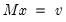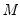Command Reference : Matrix Language Reference

 @solvesystem
Solve system of linear equations.
Syntax: @solvesystem(o, v)
m: matrix, sym
v: vector
Return: vector
Returns the vector x that solves the linear systemwhere the matrix or symis given by the argument m, and the vector is given by the argument v.
Examples
We first create a workfile, generate a random series, and create a group for estimating a trend regression.
workfile u 100
series y = nrnd
group g 1 @trend
We extract the moment matrices,
matrix xx = @inner(g)
vector xy = @transpose(@convert(g)) * @convert(y)
and solve the moment conditions to obtain regression coefficient estimates
vector b1 = @solvesystem(xx, xy)
We may compare these results to those obtained by estimation using series and the equation object:
equation eq1.ls y g
vector b2 = eq1.@coefs
and see that B1 and B2 are identical.
Cross-references